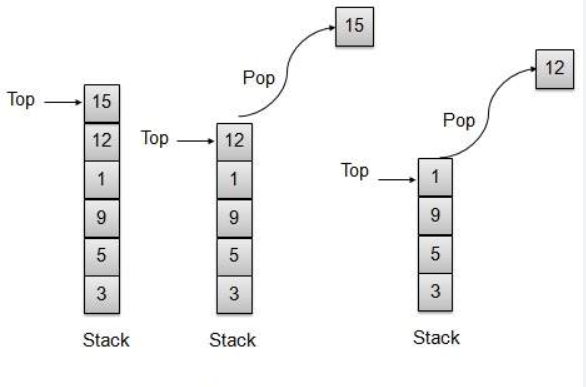# Stacks

28 January 2023

### Data Structure: Stacks

Stacks are basically dynamic data structures used in programming languages for implementation.

It follows LIFO(Last In First Out) policy, which means the element that is inserted into the stack at the last will be popped at first.Stack is linear data structures because stack is stored in the memory in a linear manner. In stacks, we can only access the element at the top of the stack, that is, the element that is pushed at last to the stack.

There are various operations that can be performed in stacks :-

1. Pop()

2. Push()

3. Top()

4. Empty()

5. Size()

Push()

Time complexity – O(1)

Since we are only pushing element so it requires constant time.

`stack<int>s;`
`// push operation is used to insert the value to the stack`

` // keep in mind that the last element inserted to stack is the top most`
` // element and will be accessed at first`
`    s.push(1);`
`    s.push(2);`
` `

Pop()

Time complexity – O(1)

Since we are only popping a single element so it requires constant time.

`stack<int>s;`
` // push operation is used to insert the value to the stack`

` // keep in mind that the last element inserted to stack is the top most`
` // element and will be accessed at first`
`    s.push(1);`
`    s.push(2);`
`    s.pop();`
`// pop is simply used to delete the last inserted value to the stack`
`// initially our stack contained 1 and 2 and now since we used pop`
`// opertion , it will contain only 1 since 2(the last most element inserted)`
`// is popped out by using pop operation`

TOP()

Time complexity – O(1)

Top element is maintained internally by using a pointer and return the value in constant time.

`int x =s.top();`
`cout<<x<<endl;`
`// top operation will return the top most element of the stack`
` `

1

Empty()

Time complexity – O(1)

Since size variable is also maintained so it will return size in O(1) time .

`bool flag = s.empty();`
`cout<<flag<<endl;`
`// empty operation will return true if stack is empty and will return`
`// false if stack is not empty`

0

Size()

Time complexity – O(1)

Since size variable is also maintained so it will return size in O(1) time .

` cout<<s.size()<<endl;`
`// it will return the size of the stack , since in this case size of`
`// stack is 1 as we have pushed 1 and 2 and then popped out 2`
` `Received Output:

1

#### Implementation of Stack

`#include "bits/stdc++.h"`
`using namespace std;`

`const int N = 1000;`
`// N is the maximum size we can push into the stack`
`int arr[N];`
`int temp=-1;`
`// this is the pointer that will help to find the top value of the stack`

`void push(int x)`
`{`
`  arr[temp+1] = x;`
`  temp++;`
`  // we increase the value of temp as we have added a value to the stack`
`}`

`void pop()`
`{`
`  // we simply reduce temp so as to perform pop operation`
`   temp--;`
`}`

`int top()`
`{`
`  // if stack is empty we will return -1e9 which means it is empty  `
`  if(temp==-1)return -1e9;`
`  //otherwise we will return the value at which temp points`
`  return arr[temp];`
`}`

`int size()`
`{`
`  //value of temp +1 returns the size`
`  return temp+1;`
`}`

`bool empty()`
`{`
`  // if temp points to -1 it means pointer is at the starting thus it is empty`
`  if(temp==-1)return true;`
`  return false;`
`}`

`int main()`
`{`
`    push(1);`
`    push(2);`

`    cout<<top()<<endl;`

`    pop();`

`    cout<<size()<<endl;`
`    cout<<empty()<<endl;`
`    `
`    return 0;`
`}`

2

1

0

1. Since stack allocates memory dynamically, we do not need to pre requisite utilize extra memory.

2. Stack is used in many complex problems where solving it by using arrays or vectors will result in much large time complexity.

• Stack memory is of limited size.

• Stack size should be defined beforehand.

• Stack overflow is another problem if too many objects are created.

• Random accessing is not possible in stack.

Key Points:

1. If stack is already empty and we use pop operation, it will result in runtime error since no element can be popped out as stack is empty. This is condition of underflow.

2. If stack is empty, then using top operation will generate random value since top of stack does not contain any value.

3. If we push a lot of elements into the stack it will result in stack overflow or over utilization of memory.

##Related Articles